# Modulus and Argument of Complex Numbers

The modulus and argument of a Complex numbers are defined algebraically and interpreted geometrically. Examples with detailed solutions are included.
A modulus and argument calculator may be used for more practice.

A complex number written in standard form as $$Z = a + ib$$ may be plotted on a rectangular system of axis where the horizontal axis represent the real part of $$Z$$ and the vertical axis represent the imaginary part of $$Z$$. The geometrical representation of complex numbers on a complex plane, also called Argand plane, is very similar to vector representation in rectangular systems of axes.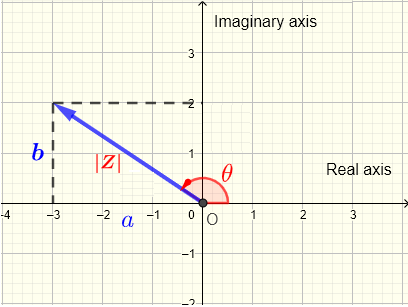The modulus of a complex number in standard form $$Z = a + ib$$ is defined by
$|z| = \sqrt{a^2 + b^2}$
and its argument $$\theta$$ is defined by
$\tan (\theta) = \left (\dfrac{b}{a} \right)$
Note
Since the above trigonometric equation has an infinite number of solutions (since $$\tan$$ function is periodic), there are two major conventions adopted for the range of $$\theta$$ and let us call them conventions 1 and 2 for simplicity.
Convention (1) define the argument $$\theta$$ in the range: $$0 \le \theta \lt 2\pi$$
Convention (2) defines the argument $$\theta$$ in the range : $$(-\pi, +\pi ]$$
The four quadrants , as defined in trigonometry, are determined by the signs of $$a$$ and $$b$$
If the terminal side of $$Z$$ is in quadrant (I) or (II) the two conventions give the same value of $$\theta$$.
If the terminal side of $$Z$$ is in quadrant (III) or (IV) convention one gives a positive angle and convention (2) gives a negative angle related by

In what follows, we use convention (1) where $$\theta$$ is in the range: $$0 \le \theta \lt 2\pi$$ but we also mention convention (2).
The graphical interpretations of the modulus $$|Z|$$ and the argument $$\theta$$ are shown below for a complex number on a complex.
The modulus $$|Z|$$ is the length of the segment representing the complex number. It may represent a magnitude if the complex number represent a physical quantity. The argument $$\theta$$ is an angle in standard position (starting from the positive direction of the axis of the real part), representing the direction of $$Z$$

If we are given the modulus $$|Z|$$ and argument $$\theta$$ of a complex number $$Z$$, then the standard form of $$Z$$ is given by
$Z = |Z| \; ( \cos \theta + i \sin \theta )$

Example 1
Plot the complex number $$Z = -1 + i$$ on the complex plane and calculate its modulus and argument.
Solution to Example 1
The complex number $$Z = -1 + i = a + i b$$ hence
$$a = -1$$ and $$b = 1$$
$$Z$$ is plotted as a vector on a complex plane shown below with $$a = -1$$ being the real part and $$b = 1$$ being the imaginary part.
The modulus of $$Z$$ , $$|Z| = \sqrt {a^2+b^2} = \sqrt {(-1)^2+(1)^2} = \sqrt 2$$ is the length of the vector representing the complex number $$Z$$.
The argument $$\theta$$ is the angle in counterclockwise direction with initial side starting from the positive real part axis.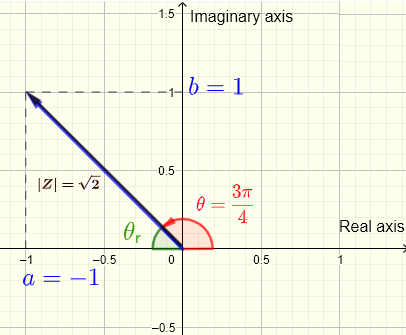$$|Z| = \sqrt {a^2 + b^2} = \sqrt {(-1)^2 + 1^2} = \sqrt {1 + 1} = \sqrt 2$$
We first need to find the reference angle $$\theta_r$$ which is the acute angle between the terminal side of $$\theta$$ and the real part axis.
$$\theta_r = \tan^{-1}\left|\dfrac{b}{a}\right| = \tan^{-1}\left|\dfrac{1}{-1}\right| =\tan^{-1} (1) = \dfrac{\pi}{4}$$
The real part of $$z$$ is negative and its imaginary part is positive, hence the terminal side of $$\theta$$ is in quadrant II (see plot of $$z$$ above).
$$\theta$$ is computed as follows:
$$\theta = \pi - \theta_r = \pi - \dfrac{\pi}{4} = \dfrac{3\pi}{4}$$
Conclusion: Modulus: $$|Z| = \sqrt 2$$ , argument: $$\theta = \dfrac{3\pi}{4}$$
Note Both conventions (1) and (2) (see definition above) give the same value for the argument $$\theta$$.

Example 2
Calculate the modulus and argument of the complex numbers:
a) $$i$$
b) $$- 2$$
c) $$- i$$
d) $$- 1 - 2i$$
e) $$1 - i$$

Solution to Example 2
a)
Let $$Z = i = a + i b$$
gives $$a = 0$$ and $$b = 1$$
Modulus: $$|Z| = \sqrt {0^2 + 1^2} = 1$$
$$tan \theta = \dfrac{1}{0} = undefined$$
An angle with terminal side on the imaginary axis has a tangent that is undefined.
It is easier to determine the argument $$\theta = \dfrac{\pi}{2}$$ from the plot of $$z = i$$ shown below.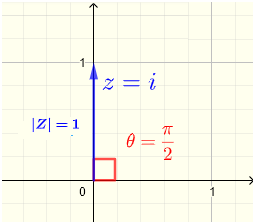Note Both conventions (1) and (2) (see definition above) give the same value for the argument $$\theta$$.

b)
Let $$Z = -2 = a + i b$$
gives $$a = -2$$ and $$b = 0$$
Modulus: $$|Z| = \sqrt {(-2)^2 + 0^2} = 2$$
$$tan \theta = \dfrac{0}{-2} = 0$$
An angle with terminal side on the real axis has a tangent equal to 0.
We can determine that the argument of $$Z$$: $$\theta = \pi$$ from the plot of $$Z = -2$$ shown below.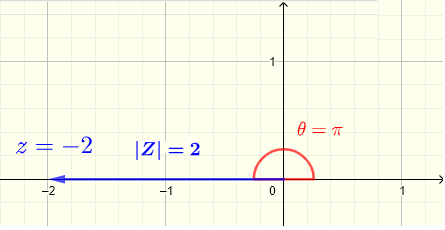Note Both conventions (1) and (2) (see definition above) give the same value for the argument $$\theta$$.

c)
Let $$Z = - i = a + i b$$
gives $$a = 0$$ and $$b = - 1$$
Modulus: $$|Z| = \sqrt {0^2 + (-1)^2} = 1$$
$$tan \theta = \dfrac{-1}{0} = undefined$$
An angle whose tangent is undefined is an angle with terminal side on the imaginary axis.
We determine $$\theta = \dfrac{3\pi}{2}$$ from the plot of $$z = - i$$ shown below.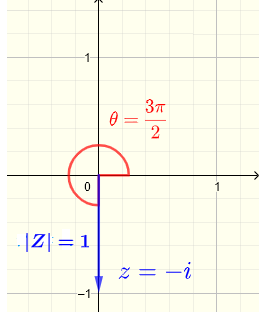Note Conventions (2) gives $$\theta = \dfrac{3\pi}{2} - 2\pi = - \dfrac{\pi}{2}$$.

d)
Let $$z = - 1 -2i = a + i b$$
gives $$a = -1$$ and $$b = - 2$$
Modulus: $$|Z| = \sqrt {(-1)^2 + (-2)^2} = \sqrt 5$$
Determine the reference angle: $$tan \theta_r = |\dfrac{-2}{-1}| = 2$$ , $$\theta_r = \arctan 2$$
The argument of $$Z$$ is: $$\theta = \pi + \theta_r = \pi + \arctan 2 \approx 4.25$$Note Convention (2) gives $$\theta = \pi + \arctan 2 - 2\pi = -\pi + \arctan 2 \approx -2.03444$$.

e)
Let $$Z = 1 - i = a + i b$$
gives $$a = 1$$ and $$b = - 1$$
Modulus: $$|Z| = \sqrt {(1)^2 + (-1)^2} = \sqrt 2$$
Determine the reference angle: $$tan \theta_r = |\dfrac{-1}{1}| = 1$$ , $$\theta_r = \dfrac{\pi}{4}$$
The argument of $$Z$$ is: $$\theta = 2\pi - \theta_r = 2\pi - \dfrac{\pi}{4} = \dfrac{7\pi}{4}$$Note Convention (2) gives $$\theta = \dfrac{7\pi}{4} - 2\pi = - \dfrac{\pi}{4}$$.

Example 3
Plot each of the complex number given by its modulus and argument and write it in standard form.
a) $$|Z_1| = 3$$ , $$\theta_1 = 0$$
b) $$|Z_2| = 4$$ , $$\theta_2 = 135^{\circ}$$
c) $$|Z_3| = 2$$ , $$\theta_3 = \dfrac{7\pi}{6}$$
b) $$|Z_4| = 2.5$$ , $$\theta_4 = 300^{\circ}$$

Solution to Example 3
The plot of all given complex numbers is shown below.Write in standard form.
a) $$Z_1 = 3\cos 0 + 3 \sin 0 i = 3$$
b) $$Z_2 = 4\cos 135^{\circ} + 3 \sin 135^{\circ} i \approx -2.88 + 2.88 i$$
c) $$Z_3 = 2\cos \dfrac{7\pi}{6} + 2 \sin \dfrac{7\pi}{6} i \approx - 1.73 - i$$
c) $$Z_3 = 2\cos \dfrac{7\pi}{6} + 2 \sin \dfrac{7\pi}{6} i \approx 1.25 - 2.17 i$$

You may also review De Moivre's Theorem Power and Root of Complex Numbers.

## Questions

1) Calculate the modulus and argument (in degrees and radians) of the complex numbers.
1. $$z_1 = - 1$$
2. $$z_2 = - 2 i$$
3. $$z_3 = -\sqrt 3 - i$$
4. $$z_4 = - 3 + 3\sqrt 3 i$$
5. $$z_5 = 7 - 7 i$$
.

2) Write in standard form the complex numbers given by their modulus and argument. .
1. $$|Z_1| = 0.5$$ , $$\theta_1 = 2.1$$
2. $$|Z_2| = 3.4$$ , $$\theta_2 = \pi/2$$
3. $$|Z_3| = 4$$ , $$\theta_3 = 0$$
4. $$|Z_4| = 12$$ , $$\theta_4 = 122^{\circ}$$
5. $$|Z_5| = 200$$ , $$\theta_5 = 5\pi/3$$
6. $$|Z_6| = 3/7$$ , $$\theta_6 = 330^{\circ}$$
.

## Solutions to the Above Questions

1)
1. $$|z_1| = 1$$ , $$\theta_1 = \pi$$ or $$\theta_1 = 180^{\circ}$$      convention(2) gives the same values for the argument
2. $$|z_2| = 2$$ , $$\theta_2 = 3\pi/2$$ or $$\theta_2 = 270^{\circ}$$      convention(2) gives: $$- \pi/2$$ or $$-90^{\circ}$$
3. $$|z_3| = 2$$ , $$\theta_3 = 11 \pi/6$$ or $$\theta_3 = 330^{\circ}$$      convention(2) gives: $$- \pi/6$$ or $$-30^{\circ}$$
4. $$| z_4 | = 6$$ , $$\theta_4 = 2\pi/3$$ or $$\theta_4 = 120^{\circ}$$      convention(2) gives same values for the argument.
5. $$| z_5 | = 2 \sqrt 7$$ , $$\theta_5 = 7\pi/4$$ or $$\theta_5 = 315^{\circ}$$      convention(2) gives: $$- \pi/4$$ or $$-45^{\circ}$$
.

2)
1. $$Z_1 = 0.5 (\cos 2.1 + i \sin 2.1) \approx 0.18 + 0.43 i$$
2. $$Z_2 = 3.4 (\cos \pi/2 + i \sin \pi/2) = - 3.4 i$$
3. $$Z_3 = 4 (\cos 0 + i \sin 0) = 4$$
4. $$Z_4 = 12 (\cos 122^{\circ} + i \sin 122^{\circ} ) \approx -6.36 + 10.18 i$$
5. $$Z_5 = 200 (\cos 5\pi/3 + i \sin 5\pi/3 )= 100-100\sqrt{3} i$$
6. $$Z_6 = (3/7) (\cos 330^{\circ} + i \sin 330^{\circ} ) = \dfrac{3\sqrt{3}}{14}- \dfrac{3}{14} i$$
.